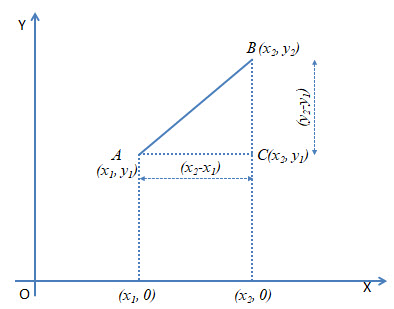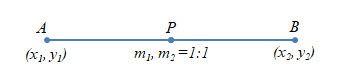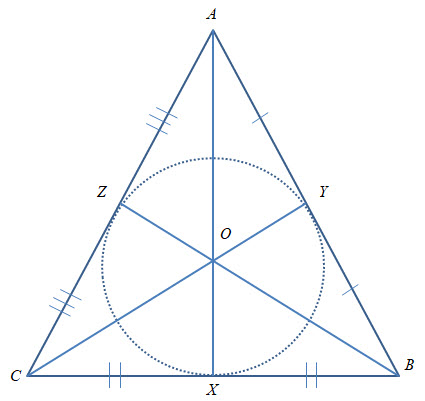This topic falls under “Coordinate Geometry“. We will learn the following topics today.

The Distance Formula (refer to the diagram as well)

Let the two given points be$A (x_1, y_1)$ and$B (x_2, y_2)$

In the diagram, you can see that the$\triangle ABC$ is a right angled triangle and$\angle C = 90^o$ . Which means that$AC^2+BC^2=AC^2$ (Pythagoras Theorem)Given$AC = x_2-x_1$ and$BC = y_2-y_1$

Hence$AB^2 = (x_2-x_1)^2 + (y_2-y_1)^2$$\Rightarrow AB = \sqrt{(x_2-x_1)^2 + (y_2-y_1)^2}$

Therefore the distance between any two points$(x_1, y_1)$ and$(x_2, y_2)$ is$= \sqrt{(x_2-x_1)^2 + (y_2-y_1)^2}$

Notes: If a point is on$x-axis$ , its ordinate is$0$ , therefore the point on$x-axis$ is taken as$(x,0)$ . Similarly, if the point is on$y-axis$ , its abscissa is$0$ , therefore the point on$y-axis$ is taken as$(0,y)$ .

Co-LinearityThree points are said to be co-linear if and only if$AC+CB=AB$ (as shown in the diagram). Which means that the distance from$A \ to \ C$ plus the distance from$C \ to \ B$ is equal to the distance from$A \ to \ B$ .

Circumcentre of  a TriangleIt is a point that is equidistant from the three vertices of a triangle. i.e. if point$P$ is equidistant from the three vertices$A, B \ and \ C$ , then$AP = BP = CP = Circumradius$ of$\triangle ABC$ .

What this means is that if a circle is drawn with$P$ as the center and any of the vertices as the radius, the circle will touch all the three vertices of the$\triangle ABC$.

Section Formula

This is used to find a point that divides a line segment joining two points in a given ratio.

Let$AB$ be the line segment. Let coordinates of$A \ be \ (x_1,y_1)$ and$B \ be \ (x_2, y_2)$ .

Let$P$ be a point dividing$AB$ in the ratio of$m_1:m_2$.$\displaystyle \text{i.e. } \frac{AP}{PB} = \frac{m_1}{m_2}$

We need to find$P(x,y)$ .

Refer to the diagram.$AR=LM=OM-OL=x-x_1$$PR=PM-RM=PM-AL=y-y_1$$PS=MN=ON-OM=x_2-x$$BS=BN-SN=BN-PM=y_2-y$

Since$\triangle APR$ and$\triangle PBS$ are similar$\displaystyle \frac{AR}{PS} = \frac{PR}{BS} = \frac{AP}{PB}$$\displaystyle \frac{AR}{PS} = \frac{AP}{PB} \Rightarrow \frac{x-x_1}{x_2-x} = \frac{m_1}{m_2}$$\Rightarrow m_2x-m_2x_1=m_1x_2-m_1x$$\Rightarrow m_1x+m_2x=m_1x_2+m_2x_1$$\Rightarrow x(m_1+m_2)=m_1x_2+m_2x_1$$\displaystyle \Rightarrow x = \frac{m_1x_2+m_2x_1}{m_1+m_2}$$\displaystyle \frac{PR}{BS} = \frac{AP}{PB} \Rightarrow \frac{y-y_1}{y_2-y} = \frac{m_1}{m_2}$$\displaystyle \Rightarrow y = \frac{m_1y_2+m_2y_1}{m_1+m_2}$$\displaystyle \text{Therefore the co-ordinates of } P = \Big( \frac{m_1x_2+m_2x_1}{m_1+m_2} , \frac{m_1y_2+m_2y_1}{m_1+m_2} \Big)$

Note: If instead of$m_1:m_2$ you used$k:1$, then the formula would become as follows:$\displaystyle P = \Big( \frac{kx_2+x_1}{k+1} , \frac{ky_2+y_1}{k+1} \Big)$

Points of TrisectionLet points$P \ and \ Q$ lie on a line segment$AB$ such that it divides the line in three equal parts i.e.$AP=PQ=QB$

Point$P$ divides the line segment$AB$ in the ratio of$1:2 \ (i.e. \ m_1 = 1, m_2 = 2)$$\displaystyle \text{Hence } P = \Big( \frac{x_2+2x_1}{3} , \frac{y_2+2y_1}{3} \Big)$

Similarly, point$Q$ divides the line segment$AB$ in the ratio$2:1 \ (i.e. \ m_1=2, m_2=1)$$\displaystyle \text{Hence } Q = \Big( \frac{2x_2+x_1}{3} , \frac{2y_2+y_1}{3} \Big)$

Midpoint FormulaLet points$P$ lie on a line segment$AB$ such that it divides the line in two equal parts i.e.$AP=PB$

Point$P$ divides the line segment$AB$ in the ratio of$1:1 \ (i.e. \ m_1 = 1, m_2 = 1)$$\displaystyle \text{Hence } P = \Big( \frac{x_2+x_1}{2} , \frac{y_2+y_1}{2} \Big)$

The centroid of a TriangleThe centroid of a triangle is the point of intersection of its medians and the centroid divides each of the medians in the ratio of$2:1$.

To find the coordinates of the centroid:

1. First, find the coordinates of the midpoint of the sides of the triangle.$(m_1:m_2=1:1)$
2. The find out the coordinates of the centroid$(m_1:m_2=1:2)$ between the vertex and the opposite midpoint.